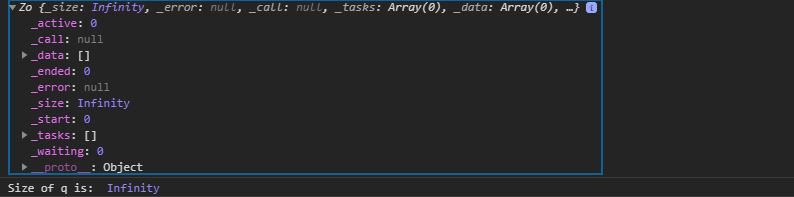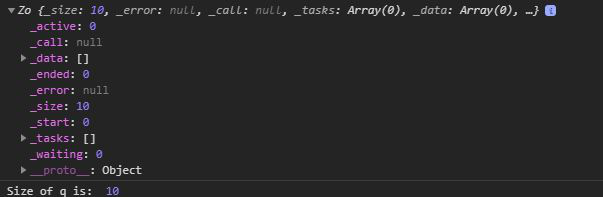Related Articles

# D3.js queue() Function

• Last Updated : 03 Aug, 2020

The d3.queue() function is used to create a queue of a specified size. If the size is not given by default it is taken as infinite.

Syntax:

``` d3.queue(size);
```

Parameters: This function accepts a single parameter mentioned above and described below:

• size: It is the optional parameter if it is not given then the size of the queue is infinite else size of the queue is the number given. The size specifies how many functions to run concurrently.

Returns: This function returns the object.

Below given are a few examples of the above function.

Example 1:  When the size of the queue is not given by default it is of infinite size.

## HTML

 ` ``<``html` `lang``=``"en"``> ``<``head``> ``    ``<``meta` `charset``=``"UTF-8"``> ``    ``<``meta` `name``=``"viewport"``            ``path1tent="``width``=``device``-width, ``                       ``initial-scale``=``1``.0"> ``    ``<``title``>D3.js queue() Function `` ``<``style``> `` ``<``body``>  ``  ``<``script` `src` `= ``"https://d3js.org/d3.v4.min.js"``> ``  `` ``  ``<``script``>``    ``let q=d3.queue()``    ``console.log(q)``    ``console.log("Size of q is: ",q._size)``  `` `` ```

Output:Example 2: When the size of the queue is given.

## HTML

 ` ``<``html` `lang``=``"en"``> ``<``head``> ``    ``<``meta` `charset``=``"UTF-8"``> ``    ``<``meta` `name``=``"viewport"``            ``path1tent="``width``=``device``-width, ``                       ``initial-scale``=``1``.0"> ``    ``<``title``>D3.js queue() Function `` ``<``style``> ``    ``h1 { ``        ``color: green; ``    ``} ``    ``svg{ ``        ``background-color: #f2f2f2; ``    ``} ``  ``.path2{ ``        ``stroke: #000; ``    ``}`` ``<``body``> ``  ``<``div``> ``    ``<``svg` `width``=``"100"` `height``=``"100"``> ``      ``<``path` `class``=``"path2"``> ``    ````  `` ``  ``<``script` `src` `= ``"https://d3js.org/d3.v4.min.js"``> ``  `` ``  ``<``script``>``    ``let q=d3.queue(10)``    ``console.log(q)``    ``console.log("Size of q is: ",q._size)``  `` `` ```

Output:Hey geek! The constant emerging technologies in the world of web development always keeps the excitement for this subject through the roof. But before you tackle the big projects, we suggest you start by learning the basics. Kickstart your web development journey by learning JS concepts with our JavaScript Course. Now at it’s lowest price ever!

My Personal Notes arrow_drop_up